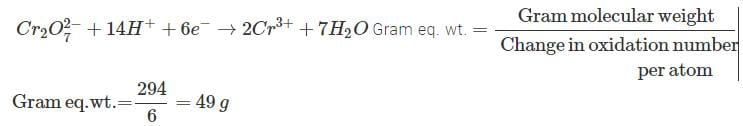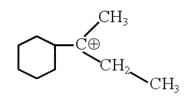# JEE Main Chemistry Mock - 5

## 25 Questions MCQ Test Mock Test Series for JEE Main & Advanced 2022 | JEE Main Chemistry Mock - 5

Description
Attempt JEE Main Chemistry Mock - 5 | 25 questions in 60 minutes | Mock test for JEE preparation | Free important questions MCQ to study Mock Test Series for JEE Main & Advanced 2022 for JEE Exam | Download free PDF with solutions
QUESTION: 1

Solution:
QUESTION: 2

Solution:
QUESTION: 3

### Which of the following sequence is correct as per Aufbau principle?

Solution:
QUESTION: 4
Which of the following is the least polar molecule?
Solution:
QUESTION: 5
Which of the following alkyl group has the maximum + I effect?
Solution:
QUESTION: 6
Consider the ground state of Cr atom (Z = 24). The numbers of electrons with the azimuthal quantum numbers, l = 1 and 2 are, respectively :
Solution:
QUESTION: 7
Alkyl groups are o- and p-directing because of
Solution:
QUESTION: 8
Which of the following factors do not favour electrovalency?
Solution:
QUESTION: 9
Glucose reacts with acetic anhydride to form
Solution:
QUESTION: 10
Which of the following shows geometrical isomerism ?
Solution:
QUESTION: 11
The co-ordination no. of a metal in a co-ordination compounds is
Solution:
QUESTION: 12
Four successive members of the first row transition elements are listed below with their atomic no. Which of them is expected to have the highest third ionisation enthalpy?
Solution:
QUESTION: 13

Which is a planar molecule?

Solution:
QUESTION: 14
In decinormal solution, CH₃COOH acid is ionised to the extent of 1.3%. If log 1.3=0.11. What is the pH of the solution?
Solution:
The dissociation of acetic acid is:

CH3COOH →  CH3COO- + H+

c – cα  cα

Concentration = 0.1 M

Degree of ionization, α = 1.3 %

c α = (0.1) * (1.3* 10^-2)

[H +]=1.3 * 10^-3

pH can be determined as:

-log (H^+) = pH = - log (1.3 * 10^-3) = 2.87

Thus the pH of the solution is 2.87
QUESTION: 15

Sodium nitroprusside reacts with sulphide ion to give a purple colour due to the formation of :

Solution:
QUESTION: 16
Which are isomers?
Solution: Isomers are molecules that have the same molecular formula, but have a different arrangement of the atoms in space. That excludes any different arrangements which are simply due to the molecule rotating as a whole, or rotating about particular bonds. For example, both of the following are the same molecule.
QUESTION: 17
Chlorination of toluene with excess of chlorine in the presence of light and heat followed by treatment with aqueous NaOH gives
Solution:
QUESTION: 18

In a polymer 30% molecules have molecular weight 20,000, 40% molecules have molecular weight 30,000 and the rest 30% molecules have molecular weight 60000. The weight average molecular weight of the polymer is

Solution:
QUESTION: 19
Electrolysis of molten sodium chloride leads to the formation of
Solution:
QUESTION: 20
In acidic medium dichromate ion oxidizes ferrous ion to ferric ion. If the gram molecular weight of potassium dichromate is 294 grams, its gram equivalent weight is....grams.
Solution:*Answer can only contain numeric values
QUESTION: 21

The sum of number of unpaired electrons in
[CoCl6]–3, [Cr(NH3)6]+3, [Zn(NH3)4]+2

Solution:
*Answer can only contain numeric values
QUESTION: 22

Sum of number of ions in aqueous solution of CrCl3.5NH3 and CrCl3.4NH3.

Solution:
*Answer can only contain numeric values
QUESTION: 23

Find the planner species out of the following species
SF2, SF4, SF6, SO2, SO3

Solution:
*Answer can only contain numeric values
QUESTION: 24

The total number of contributing structures showing hyperconjugation (involving C–H bonds) for the following carbocation is :Solution:
*Answer can only contain numeric values
QUESTION: 25

How many isomeric amines have the molecular formula C4H11N :

Solution:Use Code STAYHOME200 and get INR 200 additional OFF Use Coupon Code